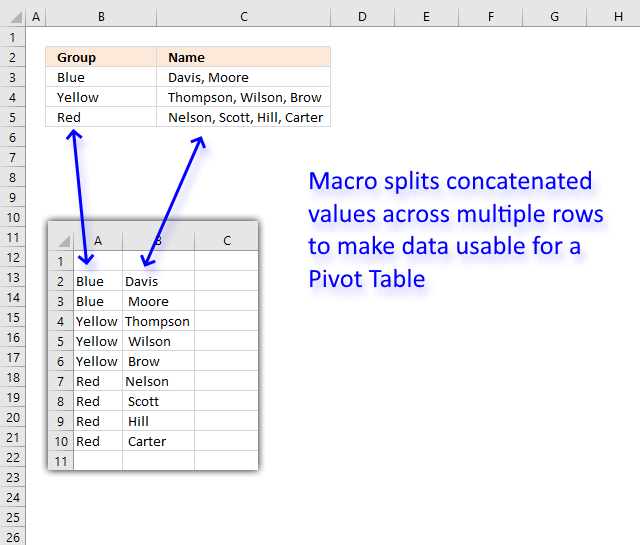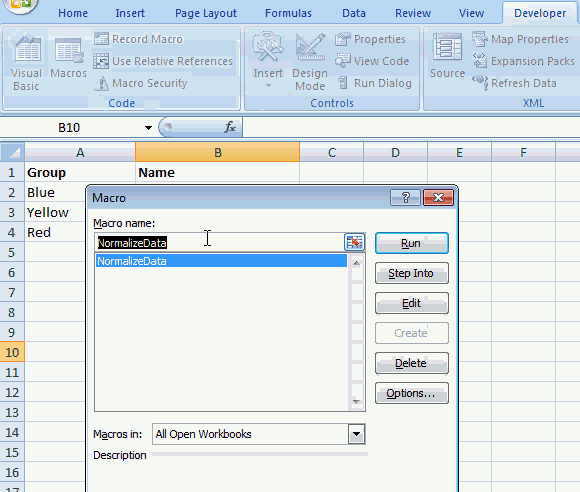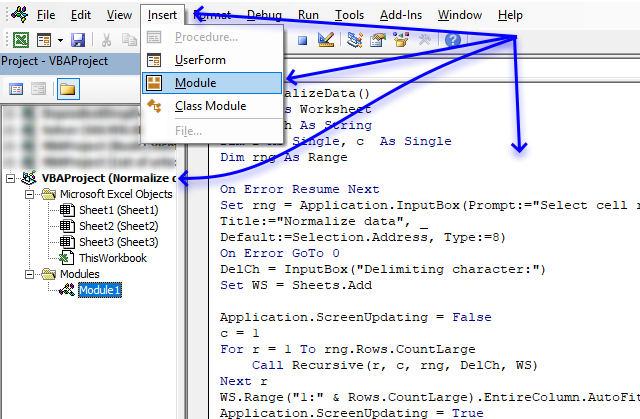Author: Oscar Cronquist Article last updated on August 28, 2019This article demonstrates a macro that allows you to rearrange and distribute concatenated values across multiple rows in order to make it possible to use the dataset in a Pivot Table.The animated image above shows how the macro works. Here are the steps to run and use the macro.

1. Press Alt+F8 to open the Macro dialog box.
2. Press with mouse on "NormalizeData" to select it.
3. Press with mouse on "Run" button to run the macro.
4. An input box appears asking for a cell range.
5. Select the cell range you want to rearrange.
6. Press with left mouse button on OK button on dialog box.
7. An input box appears asking for a delimiting character, the example shown above has a comma as a delimiting character.
8. Press with left mouse button on OK button on dialog box.
9. A new sheet is inserted and is populated with values from the cell range you selected, however, each concatenated value has now a row or record on its own. This is necessary if you want to analyze the data in a pivot table.

### VBA macros

There are two macros that make this possible, NormalizeData() and Recursive().

```'Name macro
Sub NormalizeData()

'Dimension variables and declare data types
Dim WS As Worksheet
Dim DelCh As String
Dim r As Single, c  As Single
Dim rng As Range

'Enable error handling
On Error Resume Next

'Show input box and ask for a cell range
Set rng = Application.InputBox(Prompt:="Select cell range:", _
Title:="Normalize data", _

'Disable errror handling
On Error GoTo 0

DelCh = InputBox("Delimiting character:")

'Insert a new worksheet to your workbook

'Don't show changes on screen
Application.ScreenUpdating = False

'Save 1 to variable c
c = 1

'Iterate from 1 to the number of rows in the selected cell range
For r = 1 To rng.Rows.CountLarge

'Start macro Recursive with variables r, c, rng, DelCh and WS
Call Recursive(r, c, rng, DelCh, WS)

'Continue with next row
Next r

'Change column width to fit content
WS.Range("1:" & Rows.CountLarge).EntireColumn.AutoFit

'Show changes to Excel user
Application.ScreenUpdating = True

End Sub
```

The second macro is displayed below.

```'Name macro as dimension arguments and declare data types
Sub Recursive(r As Single, c As Single, rng As Range, DelCh As String, WS As Object)

'Dimension variables and declare data types
Dim str As Variant
Dim cc As Single, ccc As Single

'Split cell using delimiting character saved to variable DelCh
str = Split(rng.Cells(r, c).Value, DelCh)

'Iterate through values in array variable str
For i = 0 To UBound(str)

'Check if variable c is equal to 1
If c = 1 Then

'Iterate from 1 to the number of columns in selected cell range
For ccc = 1 To rng.Columns.CountLarge

'Check if row number of first empty value in column ccc is larger than variable j
If WS.Cells(Rows.Count, ccc).End(xlUp).Row + 1 > j Then

'Save row number of first empty cell in column ccc to variable j
j = WS.Cells(Rows.Count, ccc).End(xlUp).Row + 1
End If

'Continue with next column
Next ccc

'If c is not equal to 1 then do the following.
Else

'Save row number of first empty cell in column c to variable j
j = WS.Cells(Rows.Count, c).End(xlUp).Row + 1
End If

'Save value in array variable str to worksheet based on row variable j and column variable c
WS.Range("A1").Offset(j - 1, c - 1).Value = str(i)

'Check if c - 1 is greater than 0 (zero)
If c - 1 > 0 Then

'Check if cell is empty based on variable j - 1 and c - 2
If WS.Range("A1").Offset(j - 1, c - 2).Value = "" Then

'Save value one row above to cell
WS.Range("A1").Offset(j - 1, c - 2).Value = _
WS.Range("A1").Offset(j - 2, c - 2).Value
End If
End If

'Check if c is equal to the number of columns in the selected cell range
If c = rng.Columns.CountLarge Then

'Check if variable i is not equal to 0 (zero)
If i <> 0 Then

'Iterate from 1 to the number of columns in the selected cell range.
For cc = 1 To rng.Columns.CountLarge - 1

'Save value in cell above to cell based on variable j - 2 and cc - 1
WS.Range("A1").Offset(j - 1, cc - 1).Value = _
WS.Range("A1").Offset(j - 2, cc - 1).Value

'Continue with next column
Next cc
End If
Else

'Run another instance of macro Recursive this time with the next column number
Call Recursive(r, c + 1, rng, DelCh, WS)
End If
Next i
End Sub
```

### Where to put the code?1. Copy both macros above.
2. Press Alt+F11 to open the Visual Basic Editor.
3. Select your workbook in the Project Explorer.
4. Press with mouse on "Insert" on the menu.
5. Press with mouse on "Module" to create a code module.
6. Paste code to code module.
Note, save your workbook with file extension *.xlsm to make sure you keep the code to the workbook.

### Get the Excel fileNormalize-data2.xlsm